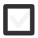# Master transitions between units within length. 1.4 km to m, dm, cm & mm. Mile, yard, feet.+1 vote
129 views

## 1 lesson

+1 vote

The meter is the base unit from smaller units are dm, cm and mm. The prefixes deci, centi and mili that we put before meter come from latin idicating deci (like decimal) - tenth part, centi - (like century) one hudreth part and mile (as mille in italian which means thousand) a one thousandth part.

For easy conversion have in mind that we use decimal system meaning step of conversion is ten. If we are converting to smaller units we multiply with than for each step of conversion because we need to get a larger number if we count in smaller units. Just as if we would count the money in cents rather than in dollars we would get a larger number though value would be the same.Picture taken from https://www.uzinggo.com/conversion-volume-measures/proportions-measurement/math-foundations-grade-6 where you can check out for more examples

If we convert from larger to a smaller unit we would multiply with ten for each step of conversion.
The exception is when we convert from meter to kilometer we devide with a thousand but that is only beacuse in between we actually have two units that we rarely use - dekameter and hectometar.

For example if we would convert 15 m to mm we would multiply with 10 to get the value in dm (150 dm) than again with 10 to get a value in cm (1500 cm) than once more with 10 to get a value in mm (15000). So for each step of conversion we multiplied with ten and since there were three steps in between we multiplied with one thousand effectively which is exactly how many milimiters there is in one meter so if we have 15 m there is 15 000 mm in it.